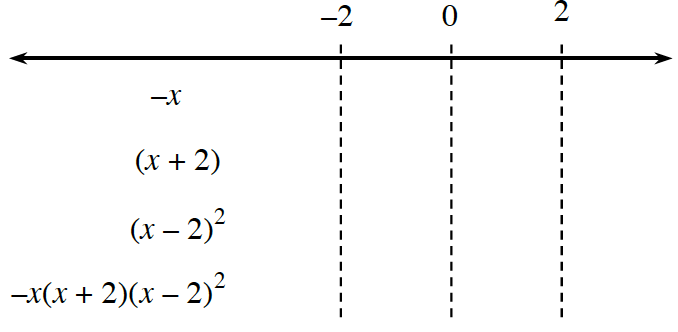### Home > PC3 > Chapter 6 > Lesson 6.2.4 > Problem6-139

6-139.

Solve each of the following inequalities.

1. $0<-x^4+2x^3+4x^2-8x$

$0<-x(x^3-2x^2-4x+8)$

$0<-x\left(x^2(x-2)-4(x-2)\right)$

$0<-x(x^2-4)(x-2)$

$0<-x(x+2)(x-2)(x-2)$

Identify the boundary points. Then complete a sign table like the one below.1. $\frac { 5 x - 10 } { 2 x ^ { 3 } + x ^ { 2 } - 50 x - 25 } \geq 0$

$\frac{5(x-2)}{x^2(2x+1)-25(2x+1)}\ge 0$

$\frac{5(x-2)}{(x^2-25)(2x+1)}\ge 0$

$\frac{5(x-2)}{(x+5)(x-5)(2x+1)}\ge 0$

Identify the boundary points then create and complete a table like the one shown in the steps for part (a).
Note: $x ≠ −5, 5, −0.5$